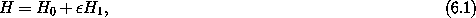# Chapter 6Canonical Perturbation Theory

 Having treated the motion of the moon about the earth, and having obtained an elliptical orbit, [Newton] considered the effect of the sun on the moon's orbit by taking into account the variations of the latter. However, the calculations caused him great difficulties ... Indeed, the problems he encountered were such that [Newton] was prompted to remark to the astronomer John Machin that ``... his head never ached but with his studies on the moon.'' June Barrow-Green, Poincaré and the Three Body Problem , p. 15

Closed-form solutions of dynamical systems can be found only rarely. However, some systems differ from a solvable system by the addition of a small effect. The goal of perturbation theory is to relate aspects of the motion of the given system to those of the nearby solvable system. We can try to find a way to transform the exact solution of this approximate problem into an approximate solution to the original problem. We can also use perturbation theory to try to predict qualitative features of the solutions by describing the characteristic ways in which solutions of the solvable system are distorted by the additional effects. For instance, we might want to predict where the largest resonance regions are located or the locations and sizes of the largest chaotic zones. Being able to predict such features can give insight into the behavior of the particular system of interest.

Suppose, for example, we have a system characterized by a Hamiltonian that breaks up into two parts as follows:where H0 is solvable andis a small parameter. The difference between our system and a solvable system is then a small additive complication.

There are a number of strategies for doing this. One strategy is to seek a canonical transformation that eliminates from the Hamiltonian the terms of orderthat impede solution -- this typically introduces new terms of order2. Then one seeks another canonical transformation that eliminates the terms of order2 impeding solution, leaving terms of order3. We can imagine repeating this process until the part that impedes solution is of such high order inthat it can be neglected. Having reduced the problem to a solvable problem, we can reverse the sequence of transformations to find an approximate solution of the original problem. Does this process converge? How do we know we can ever neglect the remaining terms? Let's follow this path and see where it goes.